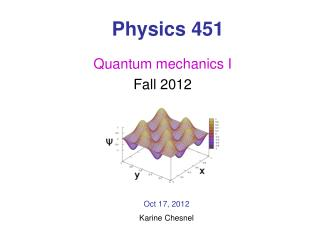Download PresentationPhysics 451

# Physics 451 - PowerPoint PPT PresentationDownload Presentation## Physics 451

- - - - - - - - - - - - - - - - - - - - - - - - - - - E N D - - - - - - - - - - - - - - - - - - - - - - - - - - -
##### Presentation Transcript

1. Physics 451 Quantum mechanics I Fall 2012 Oct 17, 2012 Karine Chesnel

2. Phys 451 Announcements • Next homework assignments: • HW # 14 due Thursday Oct 18 by 7pm • Pb 3.7, 3.9, 3.10, 3.11, A26 • HW #15 due Tuesday Oct 23 Practice test 2 M Oct 22 Sign for a problem! Test 2: Tu Oct 23 – Fri Oct 26

3. For a given transformation T, there are “special” vectors for which: is transformed into a scalar multiple of itself is an eigenvector of T l is an eigenvalue of T Quantum mechanics Eigenvectors & eigenvalues

4. Find the N roots Spectrum Quantum mechanics Eigenvectors & eigenvalues To find the eigenvalues: We get a Nth polynomial in l: characteristic equation Pb A18, A25, A 26

5. Quantum mechanics Discrete spectra Degenerate states More than one eigenstate for the same eigenvalue Gram-Schmidt Orthogonalization procedure See problem A4, application A26

6. Quantum mechanics Discrete spectra of eigenvalues 1. Theorem: the eigenvalues are real 2. Theorem: the eigenfunctions of distinct eigenvalues are orthogonal 3. Axiom: the eigenvectors of a Hermitian operator are complete

7. Quantum mechanics Continuous spectra of eigenvalues • No proof of theorem 1 and 2… but intuition for: • Eigenvalues being real • Orthogonality between eigenstates • Compliteness of the eigenstates Orthogonalization Pb 3.7

8. For real eigenvalue p: • Dirac orthonormality • Eigenfunctions are complete Wave length – momentum: de Broglie formulae Quantum mechanics Continuous spectra of eigenvalues Momentum operator:

9. Quantum mechanics Continuous spectra of eigenvalues Position operator: • - Eigenvalue must be real • Dirac orthonormality • Eigenfunctions are complete

10. but • If eigenvalues are real: • Dirac orthonormality • Eigenfunctions are complete Quantum mechanics Continuous spectra of eigenvalues Eigenfunctions are not normalizable Do NOT belong to Hilbert space Do not represent physical states

11. We measure an observable (Hermitian operator) • Operator’s eigenstates: Discrete spectrum Continuous spectrum eigenvector eigenvalue Phys 451 Generalized statistical interpretation • Particle in a given state Eigenvectors are complete:

12. Operator’s eigenstates: orthonormal Phys 451 Generalized statistical interpretation Particle in a given state • Normalization: • Expectation value

13. Phys 451 Quiz 18 If you measure an observable Q on a particle in a certain state , what result will you get? • the expectation value • one of the eigenvalues of Q • the average of all eigenvalues • A combination of eigenvalues • with their respective probabilities

14. Phys 451 Generalized statistical interpretation Operator ‘position’: Probability of finding the particle at x=y:

15. Phys 451 Generalized statistical interpretation Operator ‘momentum’: Probability of measuring momentum p: Example Harmonic ocillator Pb 3.11

16. Phys 451 The Dirac notation Different notations to express the wave function: • Projection on the position eigenstates • Projection on the momentum eigenstates • Projection on the energy eigenstates